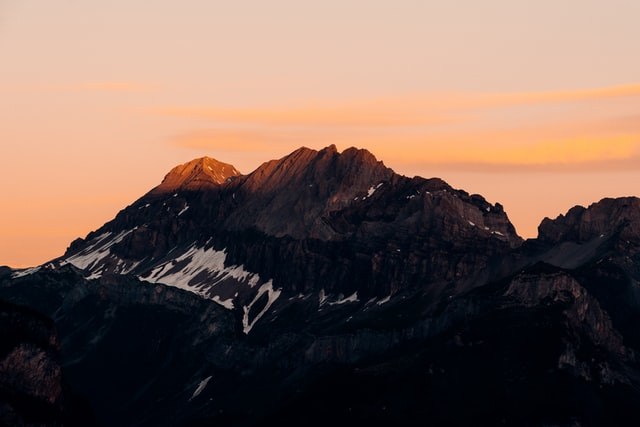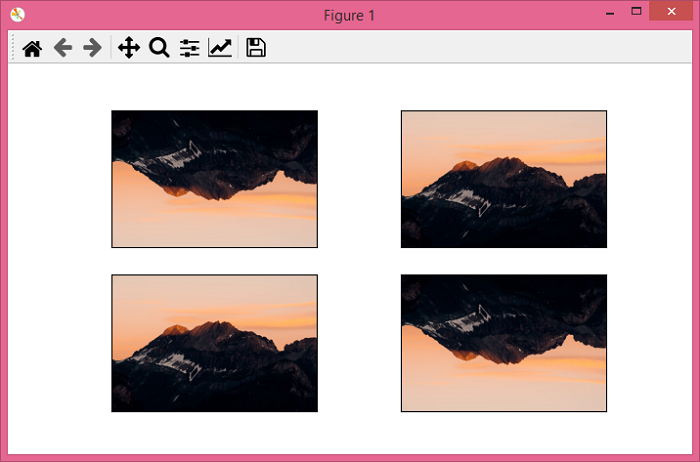# PyTorch â€“ torchvision.transforms â€“ RandomVerticalFlip()

We apply RandomVerticalFlip() transform to flip an image vertically at a random angle with a given probability. It's one of the transforms provided by the torchvision.transforms module. This module contains many important transformations that can be used to perform different types of manipulations on the image data.

RandomVerticalFlip() accepts both PIL and tensor images. A tensor image is a torch Tensor with shape [C, H, W], where C is the number of channels, H is the image height, and W is the image width.

## Syntax

torchvision.transforms.RandomVerticalFlip(p)(img)
• If p = 1, it returns the vertically flipped image.

• If p = 0, It returns the original image.

• If p is in the range (0,1), then the probability to return the vertically flipped image is p.

It returns a vertically flipped image at a random angle with a given probability p.

## Steps

One could follow the steps given below to vertically flip an image at a random angle with a given probability −

• Import the required libraries. In all the following examples, the required Python libraries are torch, Pillow, and torchvision. Make sure you have already installed them

import torch
import torchvision
import torchvision.transforms as T
from PIL import Image
• Read the input image. The input image is a PIL image or a tensor image.

img = Image.open('mountain.jpg')
• Define a transform to vertically flip the image randomly with a given probability p. Here p = 0.25 means, the chance of any input image to be vertically flipped is 25%.

transform = T.RandomVerticalFlip(p = 0.25)
• Apply the above defined transform on the input image to vertically flip the image.

vflipped_img = transform(img)
• Show the output image.

vflipped_img.show()

## Input Image

This image is used as the input file in all the following examples.## Example 1

In this program, we set p=1, so the output image will be vertically flipped image.

# import the required libraries
import torch
import torchvision.transforms as T
from PIL import Image

img = Image.open('mountain.jpg')

# define a transform with probability = 1
# to vertically flip an image
transform = T.RandomVerticalFlip(p=1)

# apply the transform on input image
img = transform(img)

# display the flipped image
img.show()

## Output

It will produce the following output −Notice that the output image is a vertically flipped image, as we set the probability p=1.

## Example 2

In this example, we set the probability p=0.25, so the chance of any image to be vertically flipped is 25%.

import torch
import torchvision.transforms as T
from PIL import Image
import matplotlib.pyplot as plt

img = Image.open('mountain.jpg')

# define a transform with probability = 0.25
transform = T.RandomVerticalFlip(p=0.25)

# save four output images applying the above transform
imgs = [transform(img) for _ in range(4)]

# display these four output images
fig = plt.figure(figsize=(7,4))
rows, cols = 2,2
for j in range(0, len(imgs)):
plt.imshow(imgs[j])
plt.xticks([])
plt.yticks([])
plt.show()

## Output

It will produce the following output −Notice that out of the four output images, at least one image is vertically flipped. You may get different number of vertically flipped images.

Updated on: 06-Jan-2022

584 Views